# How Costs Are Calculated

As mentioned in One Rate vs Two Rates, you can configure PPM Pro to use a single internal rate per role/resource, or two rates. Typically, a two-rate scenario is used when organizations need to track operational vs capital expenses. This example illustrates how costs are calculated - in summary: demand estimates are derived from the role rate, and actual amounts come from the resource rate.

We set up the example using the following:

• Role: DBA
• OpEx rate: 100, CapEx rate: 200
• Project: !Example Two Internal Rates

1. Set up Internal Rates

The screenshot below shows the internal rate table configured for OpEx and CapEx rates.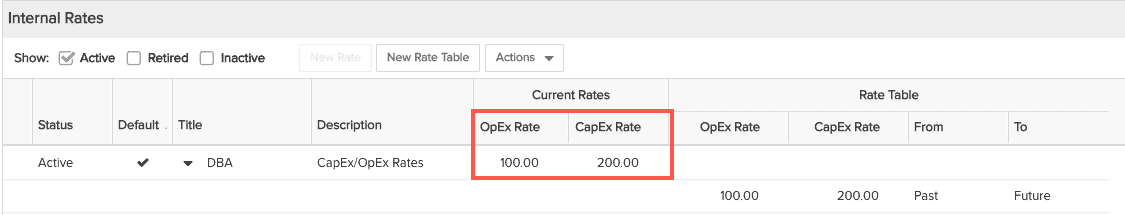2. Assign rate table to role, assign custom rate to resource

For this example the role is DBA and the resource is Ada Lovelace. After you select Assign Rate Table from the menu, select the Role radio button and select DBA. For the resource, we assigned custom rates of \$50 and \$60, for OpEx and CapEx, respectively.3. Allocate the Role

In the project Staffing screen, create an allocation for the DBA role, specify 50% for % Capitalized, and enter 40 hours. Note that you will only perform the % Capitalized step if you have enabled the Allow % Capitalization of Allocations setting in project Settings.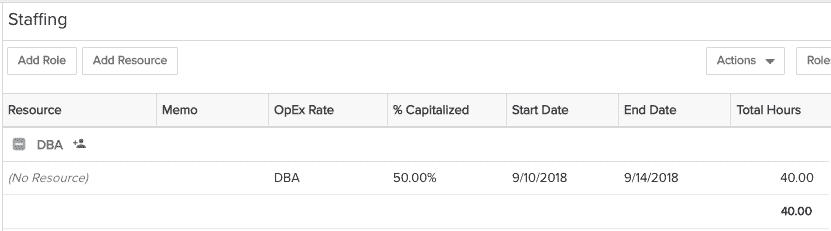4. Demand cost (Project Details section) prior to allocating resource - uses roles rates: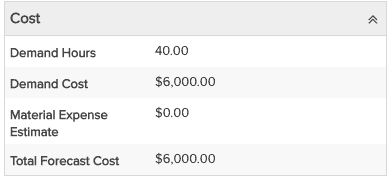5. Enter estimated hours on project Task/Expenses tab.

For each task - CapEx and OpEx, enter an estimate of 10 hours. The screenshot below shows the estimate for the OpEx task. Repeat for the CapEx task.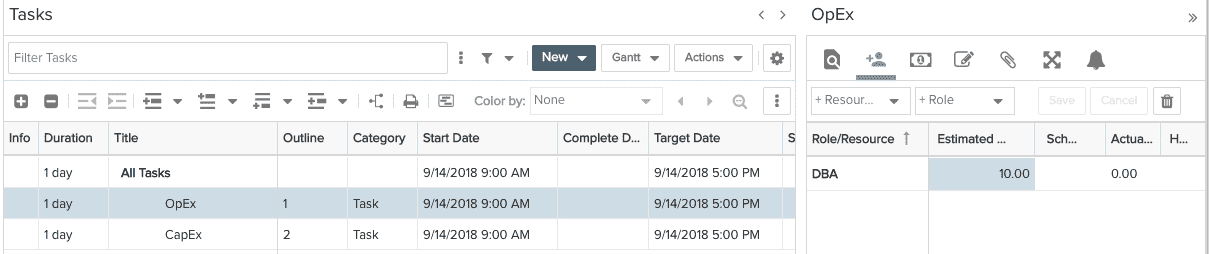6. Task Estimates/Role Rates inform the project Rollups page

Estimates are derived from role rates, as illustrated in the screenshot below. Non-Capitalized Cost (OpEx) estimate is (10 hours x \$100 = \$1000) and the Capitalized cost estimate is (10 hours x \$200 = \$2000), for a total cost estimate of \$3000.7. Staff the allocation

Once allocation is staffed, resource rates are used instead of role rate to determine demand costs.The formula for calculating demand cost with 2 rates is:

Demand Cost = ( Allocated Hours * % Capitalized * CapExRate )  + ( Allocated Hours * (100 - % Capitalized) * OpExRate)

When customer has enabled "Treat all Finances as Operational Expenses (OpEx)" or has 1 rate enabled:

Demand Cost = (Allocated Hours * Internal Rate)For this example, the cost reflects the CapEx/OpEx rates: (40 x %50) x \$50 + (40 * %50) x \$60

See here for formula if using Task-level planning.

8. Enter scheduled hours after adding the resource to the schedule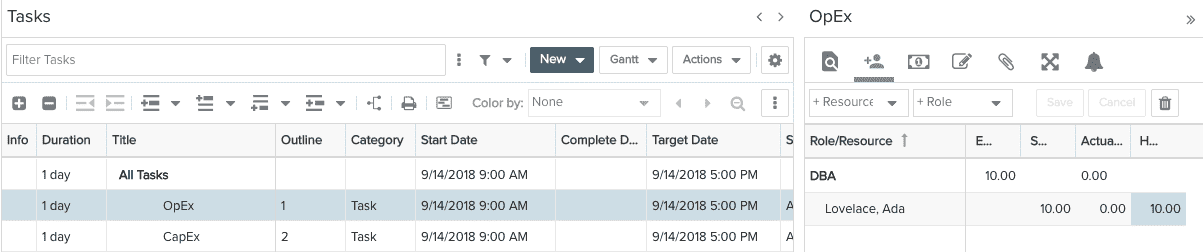9. Enter Time (actuals)

Note that you won't see the internal rates unless you are a member of the Time Manager group.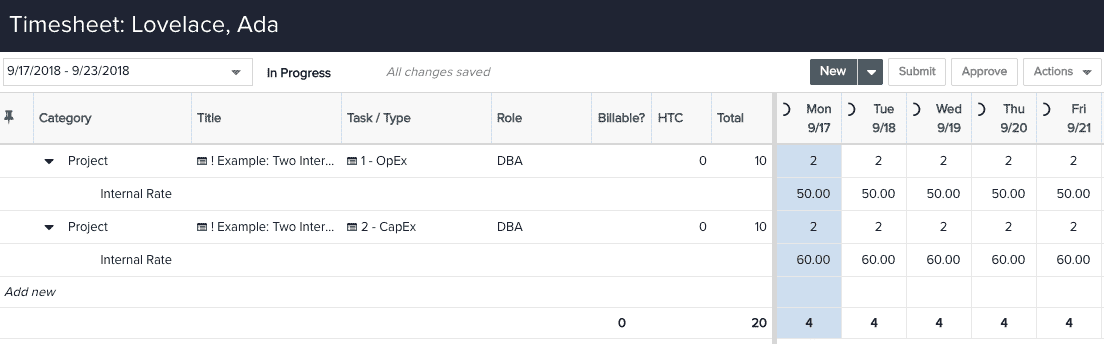10. Check project Rollups section (tasks)

Note that the Actual column reflects the actual cost derived from the resource rate.## Demand Cost Formula for Task-Level Resource Planning mode:

Conditions for using the CapEx Rate - role vs staffed resource:

• 2 rates enabled
• Project level setting "Treat all Finances as Operational Expenses (OpEx)" is Unchecked

Staffed resource:

Demand Cost = (scheduled Hours * Resource CapEx Rate) + ((Estimated Hours - Scheduled Hours) * Role CapEx Rate)

If any of the 3 conditions above are false then:

Demand Cost = (scheduled Hours * Resource OpEx Rate) + ((Estimated Hours - Scheduled Hours) * Role OpEx Rate)

Unstaffed (Role):

Demand Cost = (Estimated Hours * Role CapEx Rate)

If any of the 3 conditions above are false then:

Demand Cost = (Estimated Hours * Role OpEx Rate)